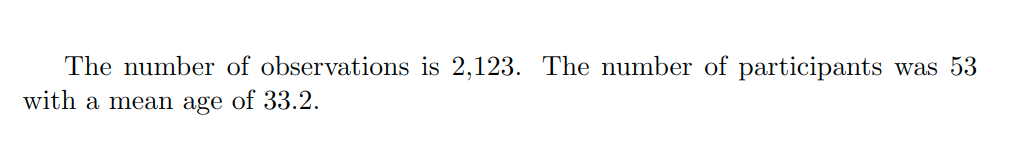# Adding dynamic computations to LaTeX documents

Recently, I’ve found myself writing up in $\LaTeX$ again, over the Rmarkdown / Quarto / knitr / pandoc / etc. ecosystem. There’s nothing fundamentally wrong with such computational notebooks, but if I had to pick one reason to stay with $\LaTeX$ it’s this: my first .tex problem sets from 2006 still compile perfectly, but I hit dependency issues with my Rmd / pandoc workflows from only a few years ago.

The one thing I did lose, though, was the ability to write placeholder variables that render into (computed) values. In Rmarkdown, you write “the mean of $x$ is r mean(x)” and it just computes and inserts the mean value into the document in place of that code snippet. If you’ve ever had to re-write numbers in your reports over and over again, you know how much of a killer feature this is for computational notebooks. However, it turns out you can do something similar in $\LaTeX$, provided you separate the dynamic insertion step from pre-computation step.

## Dynamic Insertion

Inserting values into $\LaTeX$ documents is just a matter of creating new variables using the \newcommand command in the preamble of the .tex file. Here’s a minimum working example:

\documentclass{article}

\newcommand{\nObs}{2,123}
\newcommand{\nPpl}{53}
\newcommand{\meanAge}{33.2}

\begin{document}
The number of observations is \nObs.
The number of participants was \nPpl{} with a mean age of \meanAge.
\end{document}


When the $\LaTeX$ document compiles, it replaces these custom commands with values1 they store, so the document reads:Cool, but we still have to type out the numbers in the manuscript itself, like a schmuck. To really make this trick work, we have to tell LaTeX to load these calculations from somewhere.

## Pre-computation in R (or whatever)

To make to documents dynamic, we just need to write code that saves our R calculations in the \newcommand format that $\LaTeX$ uses. Here’s the function I use, and a simple example:

This code creates a file called calcs.tex that contains something like:

\newcommand{\nObs}{2,123}
\newcommand{\nPpl}{53}
\newcommand{\meanAge}{33.2}


Note that you never have to touch these numbers yourself. R takes care of that for you.

Then, this file can be called into the main document using \input in the preamble like so:

\documentclass{article}
\input{calcs.tex}
\begin{document}
The number of observations is \nObs.
The number of participants was \nPpl{} with a mean age of \meanAge.
\end{document}


Voila! We can re-run our R scripts as a project evolves and re-generate all our figures, tables and in-text calculations. The $\LaTeX$ document will always be up to date, just like a computational Rmarkdown-style notebook.

1. Note the curly brackets in \nPpl{} are necessary to tell $\LaTeX$ to add a whitespace afterwards. ↩︎